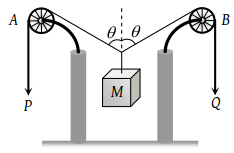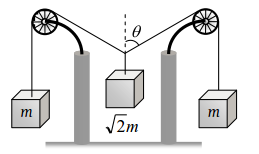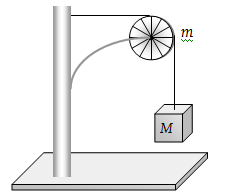Two masses m1 and m2 (m1 > m2) are connected by massless flexible and inextensible string passed over massless and frictionless pulley. The acceleration of centre of mass is

(1) ${\left(\frac{{m}_{1}-{m}_{2}}{{m}_{1}+{m}_{2}}\right)}^{2}g$

(2) $\frac{{m}_{1}-{m}_{2}}{{m}_{1}+{m}_{2}}g$

(3) $\frac{{m}_{1}+{m}_{2}}{{m}_{1}-{m}_{2}}g$

(4) Zero

Concept Questions :-

Types of forces
High Yielding Test Series + Question Bank - NEET 2020

Difficulty Level:

The mass of a body measured by a physical balance in a lift at rest is found to be m. If the lift is going up with an acceleration a, its mass will be measured as

(1) $m\left(1-\frac{a}{g}\right)$

(2) $m\left(1+\frac{a}{g}\right)$

(3) m

(4) Zero

Concept Questions :-

Pseudo force
High Yielding Test Series + Question Bank - NEET 2020

Difficulty Level:

Three weights W, 2W and 3W are connected to identical springs suspended from a rigid horizontal rod. The assembly of the rod and the weights fall freely. The positions of the weights from the rod are such that

(1) 3W will be farthest

(2) W will be farthest

(3) All will be at the same distance

(4) 2W will be farthest

Concept Questions :-

Spring force
High Yielding Test Series + Question Bank - NEET 2020

Difficulty Level:

When forces F1, F2, F3 are acting on a particle of mass m such that F2 and F3 are mutually perpendicular, then the particle remains stationary. If the force F1 is now removed then the acceleration of the particle is

(1) ${F}_{1}/m$

(2) ${F}_{2}{F}_{3}/m{F}_{1}$

(3) $\left({F}_{2}-{F}_{3}\right)/m$

(4) ${F}_{2}/m$

Concept Questions :-

Application of laws
High Yielding Test Series + Question Bank - NEET 2020

Difficulty Level:

Consider the following statement: When jumping from some height, you should bend your knees as you come to rest, instead of keeping your legs stiff. Which of the following relations can be useful in explaining the statement?

1. $\Delta \stackrel{\to }{{P}_{1}}=-\Delta \stackrel{\to }{{P}_{2}}$

2. $\Delta E=-\Delta \left(PE+KE\right)=0$

3. $\stackrel{\to }{F}\Delta t=m\Delta \stackrel{\to }{v}$

4. $\Delta \stackrel{\to }{x}\propto \Delta \stackrel{\to }{F}$

Concept Questions :-

Newton laws
High Yielding Test Series + Question Bank - NEET 2020

Difficulty Level:

A false balance has equal arms. An object weigh X when placed in one pan and Y when placed in other pan, then the weight W of the object is equal to

(1) $\sqrt{XY}$

(2) $\frac{X+Y}{2}$

(3) $\frac{{X}^{2}+{Y}^{2}}{2}$

(4) $\frac{2}{\sqrt{{X}^{2}+{Y}^{2}}}$

Concept Questions :-

Types of forces
High Yielding Test Series + Question Bank - NEET 2020

Difficulty Level:

In the arrangement shown in figure, the ends P and Q of an unstretchable string move downwards with uniform speed U. Pulleys A and B are fixed. Mass M moves upwards with a speed1.

2.

3. $\frac{2U}{\mathrm{cos}\theta }$

4. $\frac{U}{\mathrm{cos}\theta }$

Concept Questions :-

String constraint
High Yielding Test Series + Question Bank - NEET 2020

Difficulty Level:

The pulleys and strings shown in the figure are smooth and of negligible mass. For the system to remain in equilibrium, the angle θ should be(1) 0°

(2) 30°

(3) 45°

(4) 60°

High Yielding Test Series + Question Bank - NEET 2020

Difficulty Level:

A string of negligible mass going over a clamped pulley of mass m supports a block of mass M as shown in the figure. The force on the pulley by the clamp is given by(1) $\sqrt{2}Mg$

(2) $\sqrt{2}mg$

(3) $\sqrt{{\left(M+m\right)}^{2}+{m}^{2}}g$

(4) $\sqrt{{\left(M+m\right)}^{2}+{M}^{2}}g$

High Yielding Test Series + Question Bank - NEET 2020

Difficulty Level:

A pulley fixed to the ceilling carries a string with blocks of mass m and 3 m attached to its ends. The masses of string and pulley are negligible. When the system is released, its centre of mass moves with what acceleration

(1) 0

(2) g/4

(3) g/2

(4) –g/2